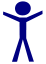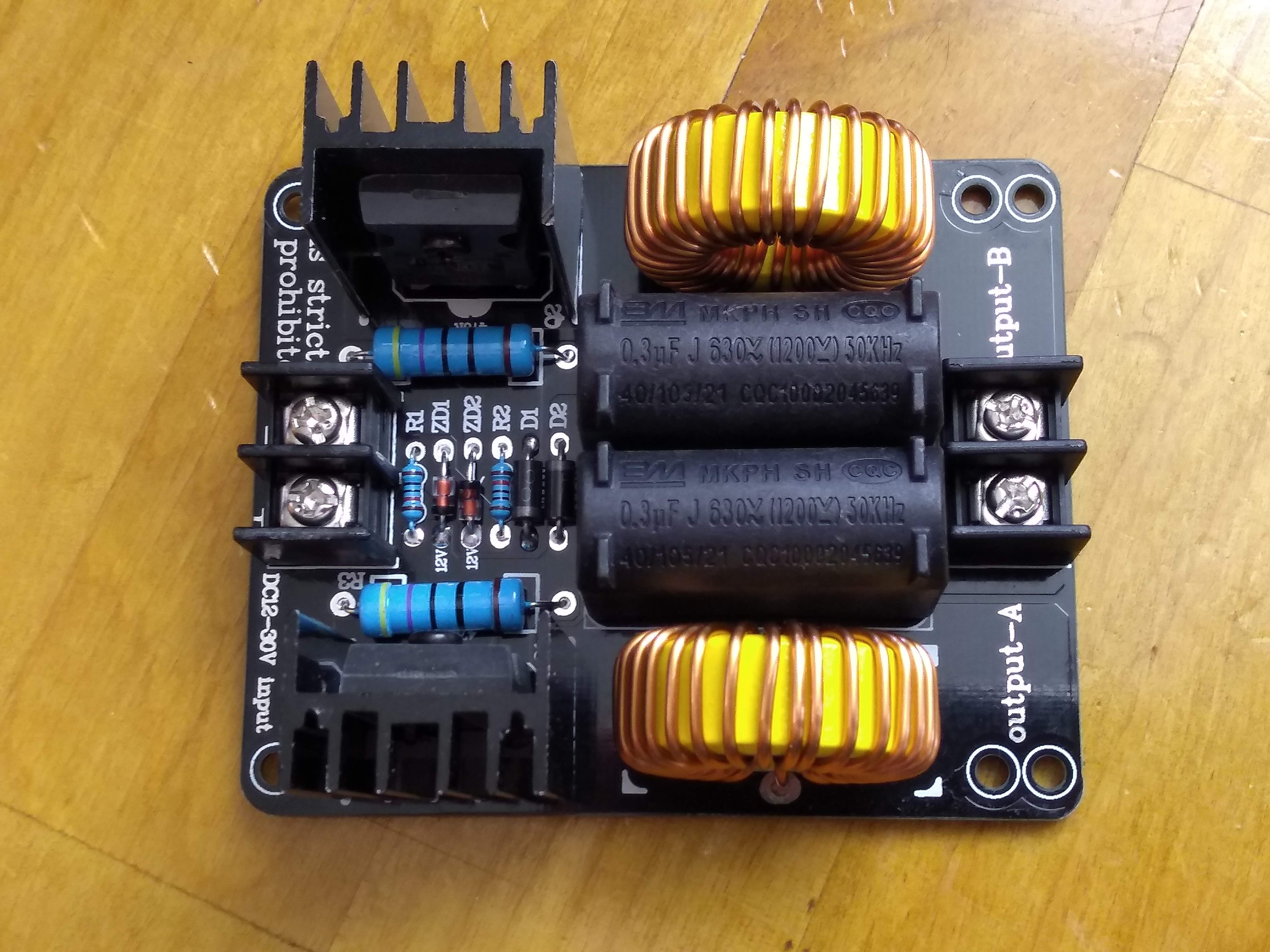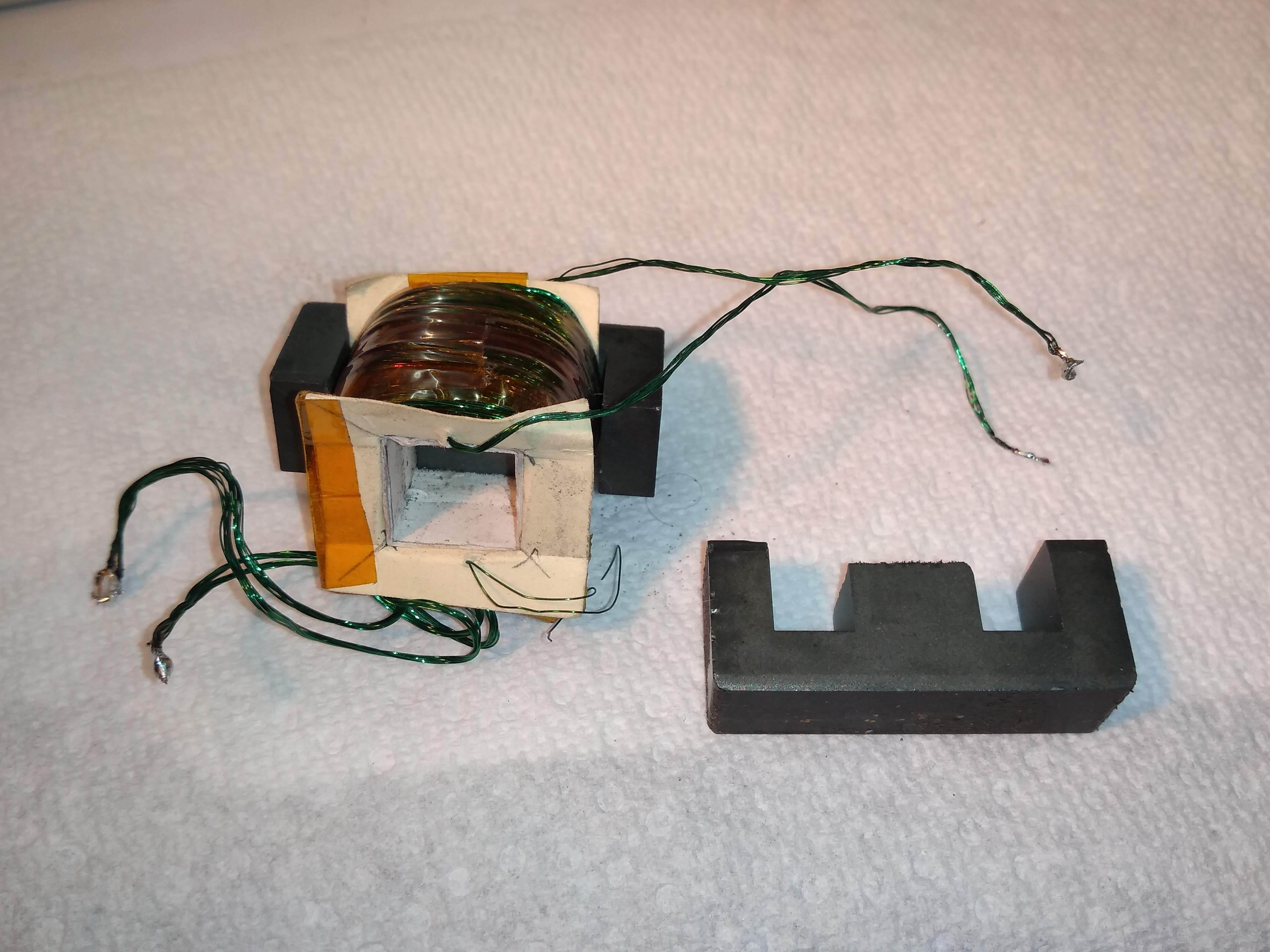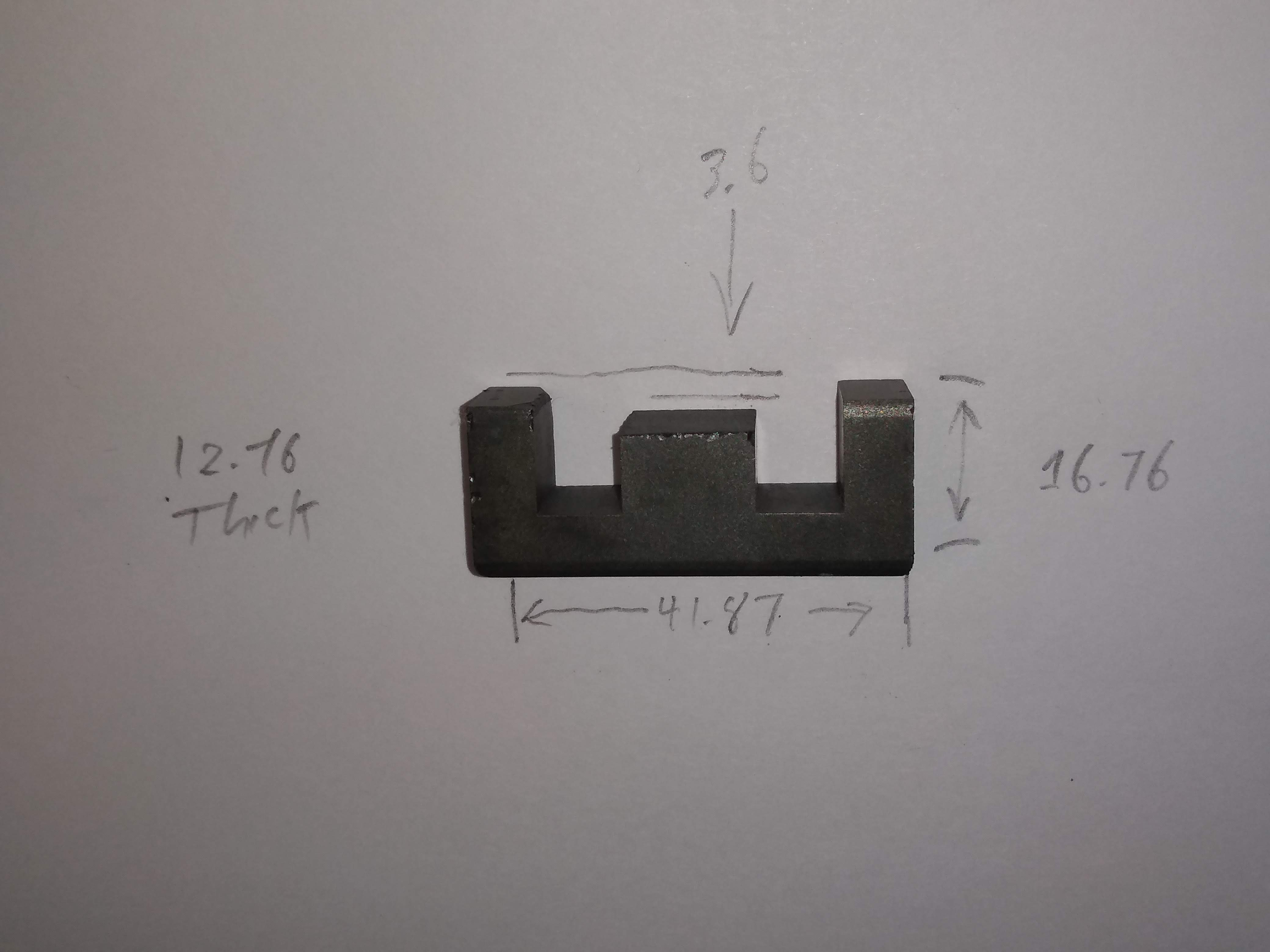#The Human Condition

### Site Tools

wiki:user:ram:vacuum:dc_supply

# DC Supply

I made a high voltage DC supply which people may be interested in. It uses an Ebay Chinese ZVS resonant driver and a custom transformer, which then feeds a bridge rectifier and output inductor. At 35 V input the no-load output is 1 kV. I ran it for a while at 400V 0.375A with no difficulties (150W). The transformer starts to get hot at that current, but with the fan it can probably run indefinitely. Efficiency is about 85%.

There a switch to direct output to one of two BNC connectors, and terminals for a current meter. Also visible is a buck converter board which drops the input down to 12V for the fan.

## The ZVS inverter

The key active component is the ZVS inverter board which converts the DC input to an 87 kHz sine wave. One of the big virtues of this approach is that you can get the boards on Ebay for $20 or less each. Fancier boards with fan and current limit are more like \$50. You can google this as the Mazilli driver. The circuit here is very similar to my board bought on Ebay.

The Ebay board uses dual feed inductors so that it does not need a center-tapped load (only two output connections). Also, there are two component changes: the IRFP250N is changed to IRFP260N (which has 1/2 the on resistance), and the resonant capacitor is implemented as two 0.3 uF 1200V caps rather than a series parallel arrangement.

This is a photo of the inverter board as purchased:I modified the board to put the output capacitors in series rather than parallel by desoldering the capacitors and flipping them over, adding heavy wire to replace the unused PCB traces. This reduces the capacitance by 4x, doubling the resonant frequency. This was necessary because of the fairly large inductance of the transformer (which I had already built some time ago). Efficiency would probably improve if the operating frequency was further increased by changing the transformer inductance and tank capacitance, since the feed inductors are running much warmer than everything else (core losses are high).

### Resonant self-oscillation

The really nice thing about the Mazilli ZVS inverter is that it is a self-oscillating resonant inverter with output power up into the 100's of watts. Resonant means that the load (here a transformer) is part of a LC resonant circuit which is run at the resonant frequency. Running at resonance virtually eliminates switching losses in the inverter by turning the MOSFETs on/off at a time in the cycle when either the voltage across the switch or the current through the switch is zero. This can be done because the LC resonance causes the output to oscillate or ring, creating zero crossings which are no-loss switching opportunities. This design is Zero Voltage Switched (ZVS).

Self-oscillating means that by means of pure analog magic, the circuit figures out what the resonant frequency is. You can stick a very large variety of output loads on the inverter, and it will just “work”, which is user-friendly. In production resonant converters it is more common to use a driven design where there is a separate oscillator that gets tuned to the resonant frequency by other auxiliary circuits. This avoids the startup problems of the Mazilli ZVS design.

### Inverter startup

The big flaw with this inverter is that it does not self-start. It requires an abrupt turnon of the input power in order for the oscillation to start, and the oscillation can stop if the output load is too high (arcing or a short). Being stopped might not seem so bad except that when the inverter is stopped both transistors turn on, shorting the input power supply. This is basically a hobby grade circuit, and can't be used reliably without some external protection. At a minimum, you need to run it from a supply with output current limiting, so that nothing blows immediately when the inverter stops. Even with the current limiting, the inverter may burn out if it is powered for a long time when not oscillating, since all of the input power is being dissipated in the inverter itself, rather than in the load.

### Input supply

I have been running this inverter off of a CC/CV bench supply, which provides current limiting, and also lets you roughly control the output current. The inverter is spec'd for 12V to 30V input. There is some room to push the upper voltage limit, I've gone up to 35V and nothing blew. The page linked above suggests 10-40 V input.

### Transformer

The transformer is the trickiest component here. High-frequency power transformers are basically never off-the-shelf components because there are too many variables that need to be optimized simultaneously: input voltage, output voltage, operating frequency, mechanical shape, efficiency. Designing a resonant transformer adds an additional constraint: the primary must have a certain resonant frequency. This balancing act is important to get a decent level of efficiency in the transformer. For hobby use the efficiency concern is mainly keeping the transformer from smoking, rather than reducing your electric bill.

One of the most common uses for these ZVS boards is to drive a flyback transformer (as used in CRT monitors). This will give you 10's of kV output at 10's of mA, and the flyback usually has an integrated HV rectifier also. A flyback transformer would be a good place to start if you want kV outputs. The typical ZVS/flyback setup seems to be optimized for “how long a spark can I make” (operating only a short time to avoid transformer burnout). If you want to operate continuously you will need to settle for lower output.

amazing1.com (Information Unlimited) has some HV transformers that look nice, at a reasonable price: High Voltage Transformers.

But if you want a supply for plasma discharges such as glowbar, hollow cathode, and magnetron, then you need higher current at lower voltage. So, custom transformer. The usual goal of transformer design is to get the smallest transformer which can handle the desired power without getting too hot. Losses in a transformer are divided into core losses and copper losses. A basic principle of transformer design is to make the core and copper losses roughly equal. Other effects may shift you away from equality, but you can make a blunder which makes either the core or copper losses dominate. If you are using all of the winding area in the core, then reducing one loss will increase the other. For example, using more turns of thinner wire will reduce core losses but increase copper losses.

Key points in my transformer design:

1. Large air gap. You need many mm of air gap in the core to permit you to wind many turns on the primary without pushing the resonant frequency too low.
2. Litz wire. Rather than using a thick wire to carry the current, use many thin wires twisted together (in parallel). This really helps to reduce eddy current losses in windings operating over the 20 kHz – 200 kHz range.
3. Layer winding. In a high-voltage winding it helps to prevent insulation breakdown if you wind in neat layers, where each layer is separated by an extra insulator. I used paper and Kapton tape.

Here is a view with the transformer taken apart:#### Core

This drawing shows the core measurements:I got this part surplus, so don't know the specs, but this is an standard 0.5 inch E-core, dimensions are very close to the Amidon ea-77-500, and it is virtually certain that the material is a power ferrite similar to Amidon #77, with initial permeability $\mu_0$ near 2000. Also, the permeability becomes less important as the gap increases. There are some roughly similar cores in stock on Digi-key. Buy a bobbin (coil form) if you can. I made my own from paper.

The total core gap is 2x this (7.2 mm) because each part is gapped. I gapped the cores by using a bench grinder with a silicon carbide wheel, but this is kind of a pain because the core material is not strong and tends to break or chip. It may give a bit higher losses, but it is much easier to gap the core by using a non-conductive spacer on each of the three core legs. A few layers of (non-copper clad) PCB material works well. If using spacers you would use the half-space (3.6 mm), since each magnetic circuit has two spacers.

#### Windings

I reverse engineered the windings by measuring the primary/secondary inductances. The turns ratio is the square root of the inductance ratio, 7.6, so is known with fair confidence. I estimated the primary turns (the primary is on top, so I could count layers), then used this to derive the secondary turns.

Inductance L DC resistance Litz size n
Primary 27 uH 0.080 Ohm 14/33 60
Secondary 1.57 mH 1.45 Ohm 5/33 456

The coil is layer-wound, with each layer separated by 2-3 layers of ordinary printer paper and a wrap of Kapton (polyimide) tape. The paper is mainly to provide a flat surface for the next layer, though it does add electrical insulation too, especially if you varnish impregnate it.

#### Litz Wire

The transformer is wound with homemade twisted Litz wire with 33 Ga strands. 14/33 means 14 strands of 33 ga. I used 33 ga because I have a big spool. You would not want it much coarser than that, finer is usable if you increase the number of strands. You can easily make this yourself by wrapping wire between two posts separated by the length of Litz that you need (eg. clamps attached to the backs of two chairs). After you have laid down the desired number of strands, remove one end and twist it using an electric drill. There is also a variety of Litz wire on Ebay.

### Output characteristics

The ZVS inverter is nice for driving a plasma load because it inherently has a high output impedance (like a current source). A DC plasma discharge always requires a higher voltage to start, and then you need to back off to a lower voltage for running.

Once started, the discharge has a “negative resistance” characteristic, which means that as you increase the current, the discharge voltage decreases. If you tried to drive the discharge with a perfect voltage source (zero impedance) then the output current would run away to infinity (i.e. something blows out).

## Tank design

The transformer design is constrained by being part of a resonant “tank” circuit. In particular, $Z_0 = \sqrt{L/C} = 13.4 \Omega$. The loaded $Q$ of the tank is determined by the ratio $Z_L/Z_0$, where $Z_L$ is the load impedance as reflected back through the transformer. If we ignore the effect of the rectifier, then and output of 0.375A at 400V is $1 k\Omega$, scaled by $(n_{pri}/n_{sec})^2$ gives $Z_L = 17.3 \Omega$, or $Q = 1.29$. If $Z_L < Z_0$ then the oscillator can't run, so this is probably near the maximum load. But if the $Q$ is too high then there is a lot of resonant current circulating the tank, increasing losses. So the combination of the desired load impedance together with the operating frequency determine the values of $L$ and $C$. This is why it is necessary to reduce the transformer inductance by gapping the core.

## Rectifier and switching

The rectifier is a bridge, where each “diode” is two BYT56MV in series. This is a 1 kV avalanche rated 3A diode with 100 ns recovery time. At first I used an 0.5 A 2 kV diode, and they kept burning out. The peak current in the diode is much higher than the average current because the conduction time is fairly short (output is mostly supplied by the capacitor). For this reason, do not use a larger output filter capacitor; this increases current stress on the diodes. A polypropylene (PP) dielectric capacitor is recommended for the output filter because it has lower losses than polyester. The two resistors and neon lamp serve dual purpose as a HV indicator and bleeder to discharge the output capacitor.

At first I tried having no output capacitor, but I had trouble getting the discharge started. With the capacitor the output is the peak voltage, while the plasma is perhaps responding to more like the average.

The insulated binding posts are connected to the rectifier output. Connect the voltmeter between these two terminals, and the ammeter between whichever side is grounded and the ground terminal. The “polarity jumper” is the wire visible, which is connected to the non-grounded side of the rectifier output. This allows you to switch the output polarity by moving the jumper and ammeter connections.

The jumper feeds the output inductor, which is placed in series with the output to increase the output impedance at high frequencies. This inductor is made by many turns of 24 ga wire on a 56 mm diameter x 36 mm height ferrite bobbin core. The inductance is 15 mH and DC resistance is 2.5 Ohm. This inductor is random wound rather than layer wound, which is not ideal from a voltage withstand perspective, since the coil sees the full output voltage when there is an arc. I didn't experiment with the output inductor because I already had this lying around, and it “worked”. My feel is that the inductance could likely be several times lower, while it is probably impossible for it to too large.